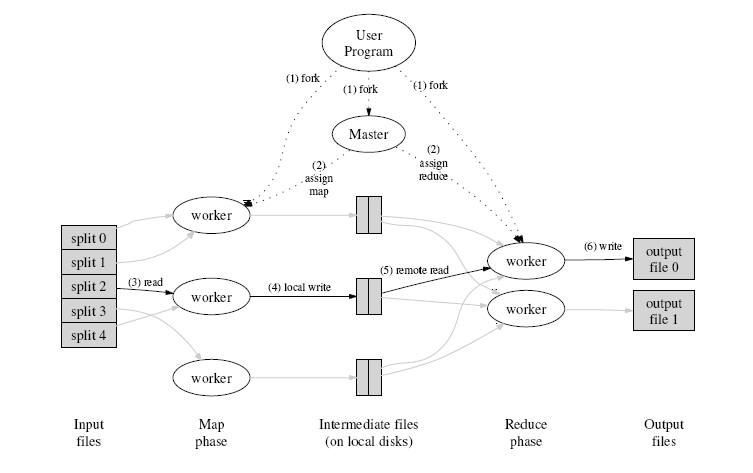In :
from IPython import display
display.Image('https://mikecvet.files.wordpress.com/2010/07/mrfigure.png')

Out:In :
a = [1, 2, 3]
b = [4, 5, 6, 7]
c = [8, 9, 1, 2, 3]
L = map(lambda x:len(x), [a, b, c])

# L == [3, 4, 5]
N = reduce(lambda x, y: x+y, L)
# N == 12
# Or, if we want to be fancy and do it in one line
N = reduce(lambda x, y: x+y, map(lambda x:len(x), [a, b, c]))

In :
N

Out:
12
In :
import sys
from multiprocessing import Pool

def sanitize(w):
"""
If a token has been identified to contain
non-alphanumeric characters, such as punctuation,
assume it is leading or trailing punctuation
and trim them off. Other internal punctuation
is left intact.
"""
# Strip punctuation from the front
while len(w) > 0 and not w.isalnum():
w = w[1:]

# String punctuation from the back
while len(w) > 0 and not w[-1].isalnum():
w = w[:-1]

return w

"""
Load the contents the file at the given
path into a big string and return it.
"""
word_list = []
f = open(path, "r")
for line in f:
word_list.append (line)

# Efficiently concatenate Python string objects
return (''.join(word_list)).split ()
def chunks(l, n):
"""
A generator function for chopping up a given list into chunks of
length n.
"""

for i in xrange(0, len(l), n):
yield l[i:i+n]

def tuple_sort (a, b):
"""
Sort tuples by term frequency, and then alphabetically.
"""
if a < b:
return 1
elif a > b:
return -1
else:
return cmp(a, b)

In :
def Map(L):
"""
Given a list of tokens, return a list of tuples of
titlecased (or proper noun) tokens and a count of '1'.
Also remove any leading or trailing punctuation from
each token.
"""
results = []
for w in L:
# True if w contains non-alphanumeric characters
if not w.isalnum():
w = sanitize(w)
# True if w is a title-cased token
if w.istitle():
results.append ((w, 1))
return results

def Partition(L):
"""
Group the sublists of (token, 1) pairs into a term-frequency-list
map, so that the Reduce operation later can work on sorted
term counts. The returned result is a dictionary with the structure
{token : [(token, 1), ...] .. }
"""
tf = {}
for sublist in L:
for p in sublist:
# Append the tuple to the list in the map
try:
tf[p].append (p)
except KeyError:
tf[p] = [p]
return tf

def Reduce(Mapping):
"""
Given a (token, [(token, 1) ...]) tuple, collapse all the
count tuples from the Map operation into a single term frequency
number for this token, and return a final tuple (token, frequency).
"""
return (Mapping, sum(pair for pair in Mapping))

In :
# Load file, stuff it into a string


In :
def compute_with_cores(cores=1):
# Build a pool of 8 processes
pool = Pool(processes=cores)

# Fragment the string data into 8 chunks
partitioned_text = list(chunks(text, len(text) /cores))

# Generate count tuples for title-cased tokens
single_count_tuples = pool.map(Map, partitioned_text)

# Organize the count tuples; lists of tuples by token key
token_to_tuples = Partition(single_count_tuples)

# Collapse the lists of tuples into total term frequencies
term_frequencies = pool.map(Reduce, token_to_tuples.items())

# Sort the term frequencies in nonincreasing order
term_frequencies.sort (tuple_sort)

for pair in term_frequencies[:3]:
print pair, ":", pair

In :
%%timeit
compute_with_cores(1)

I : 7182
The : 7116
He : 2309
I : 7182
The : 7116
He : 2309
I : 7182
The : 7116
He : 2309
I : 7182
The : 7116
He : 2309
1 loops, best of 3: 1.81 s per loop

In :
%%timeit
compute_with_cores(4)

I : 7182
The : 7116
He : 2309
I : 7182
The : 7116
He : 2309
I : 7182
The : 7116
He : 2309
I : 7182
The : 7116
He : 2309
1 loops, best of 3: 1.6 s per loop

In :
%%timeit
compute_with_cores(8)

I : 7182
The : 7116
He : 2309
I : 7182
The : 7116
He : 2309
I : 7182
The : 7116
He : 2309
I : 7182
The : 7116
He : 2309
1 loops, best of 3: 1.85 s per loop